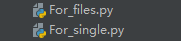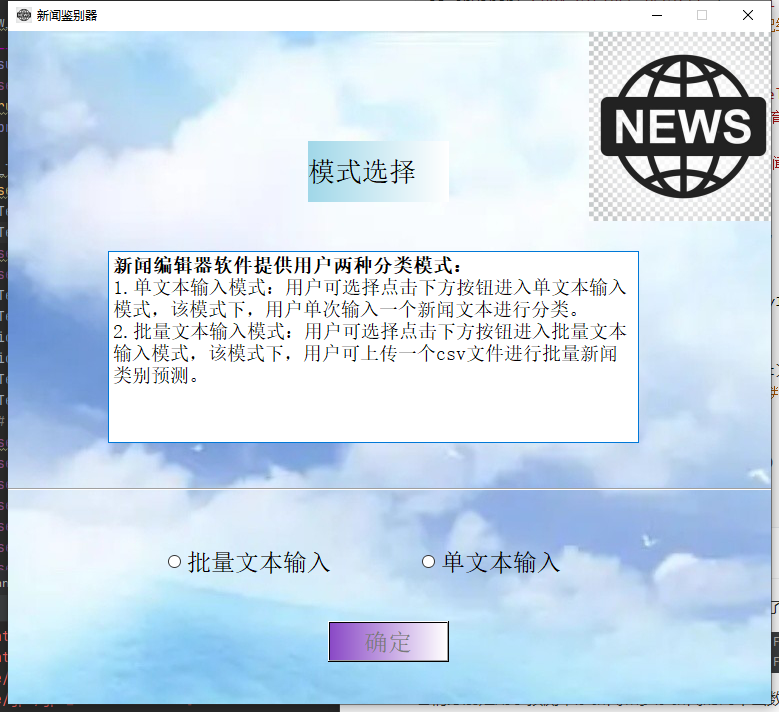``````model.add(Embedding(len(vocab) + 1, 256, input_length=300))
# 使用Embedding层将每个词编码转换为词向量
``````

``````import tensorflow as tf
from tensorflow.keras.preprocessing.sequence import pad_sequences
import os
import pandas as pd
import jieba
import pickle
import numpy as np

cw = lambda x: list(jieba.cut(x))  # jieba分词器，对输入文本分词时的必备工具
os.environ["CUDA_VISIBLE_DEVICES"] = "-1"  # 为了detect程序的稳定性，还是用cpu进行计算
fr = open('tokenizer', 'rb')  # 打开之前已经保存好的词典映射文件
fr.close()
model = tf.keras.models.load_model("model_save/model_checkpoints")  # 加载模型
tags = ['体育', '军事', '娱乐', '房产', '教育', '汽车', '游戏', '科技', '财经']
while True:
y1 = input("------------\n请输入一段新闻：(输入q退出)")
if y1 == 'q':
break
y1 = pd.DataFrame(y1, columns=["a"], index=['b'])  # 处理输入数据
y1 = y1['a'].apply(cw)
y1 = str(y1['b'])
y2 = tokenizer.texts_to_sequences([y1])
y3 = pad_sequences(y2, maxlen=300)
result = model.predict(y3)
print('置信指数：' + str(np.std(result)))  # 计算置信指数（方差）
if np.std(result) < 0.25:  # 根据方差判定是否需要相信预测结果
print('该新闻类别为其他')
else:
pred = tf.argmax(result, axis=1)
c = pred.__int__()
print('该新闻类别为' + tags[c])
``````

## 简单的UI界面#